# RS Aggarwal Solutions for Class 7 Maths Chapter 21 Collection and Organisation of Data (Mean, Median and Mode)

RS Aggarwal Solutions for Class 7 Maths Chapter 21 – Collection and Organisation of Data (Mean, Median and Mode) are available here. Maths is a subject which requires understanding and reasoning skills with logic. Along with this, it also requires students to practice Maths regularly. Students of Class 7 are suggested to solve RS Aggarwal Class 7 Solutions to strengthen the fundamentals and be able to solve questions that are usually asked in the examination.

The main aim is to help students understand and crack these problems. We at BYJU’S have prepared the RS Aggarwal Solutions for Class 7 Maths Chapter 21 wherein problems are solved step by step with detailed explanations. Download pdf of Class 7 Chapter 21 in their respective links.

## Download PDF of RS Aggarwal Solutions for Class 7 Maths Chapter 21 – Collection and Organisation of Data (Mean, Median and Mode)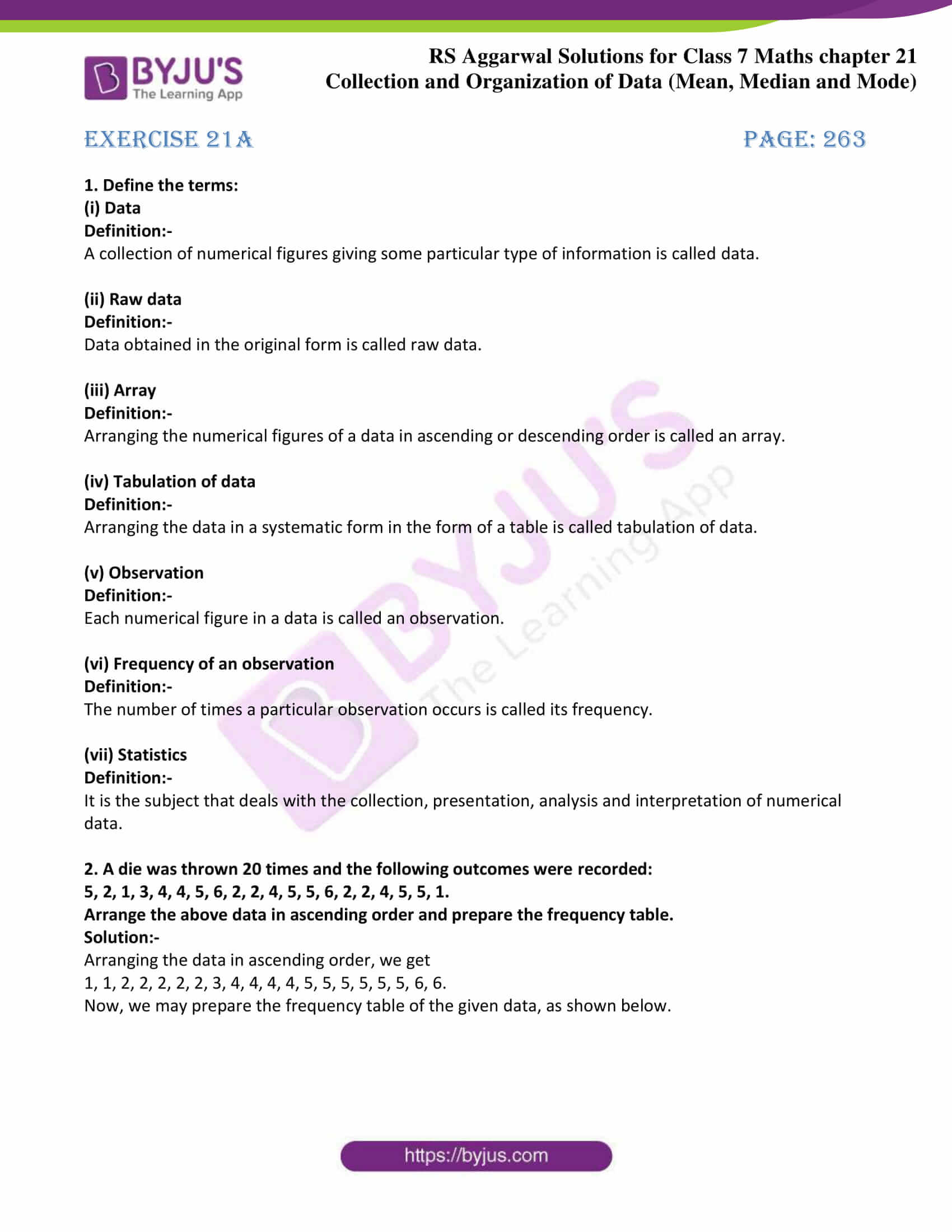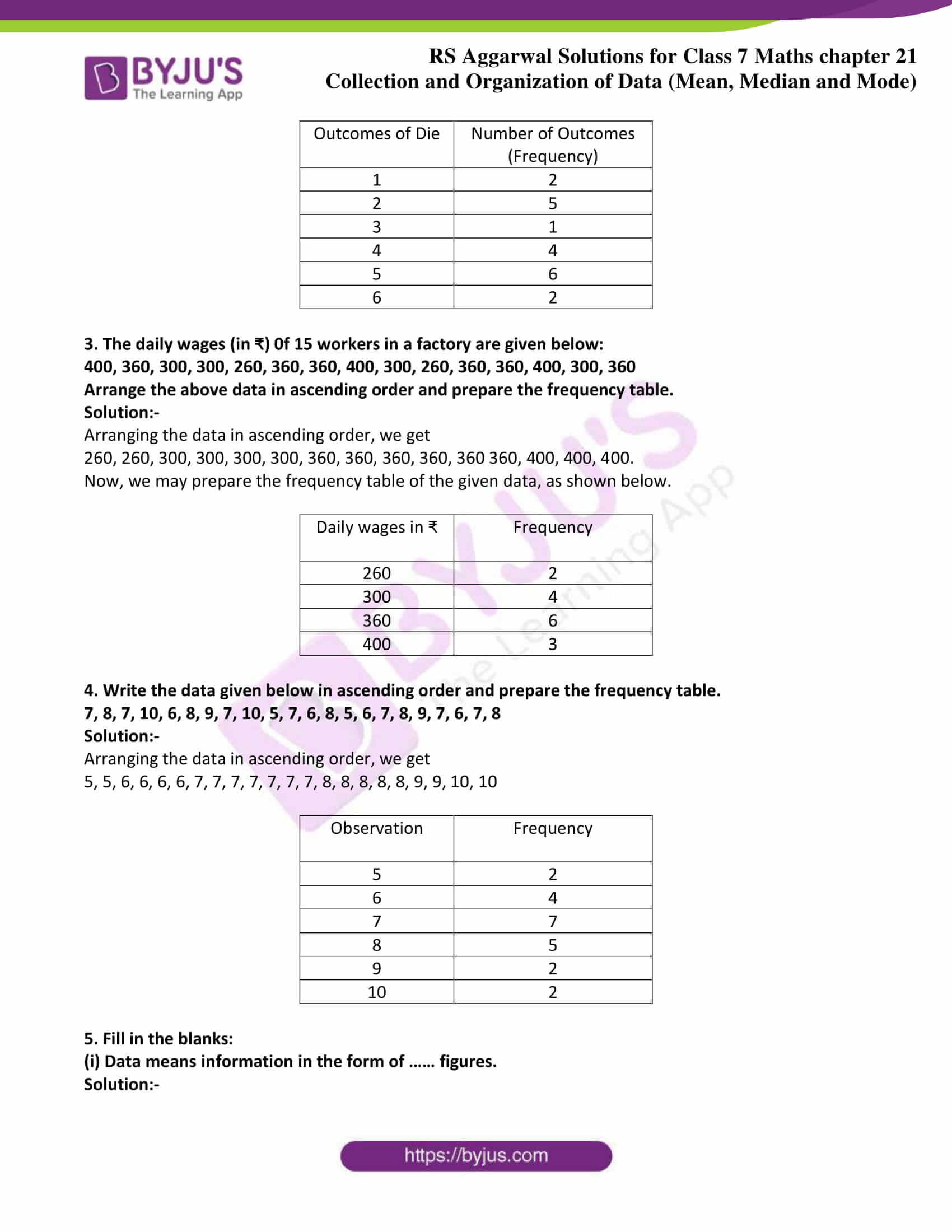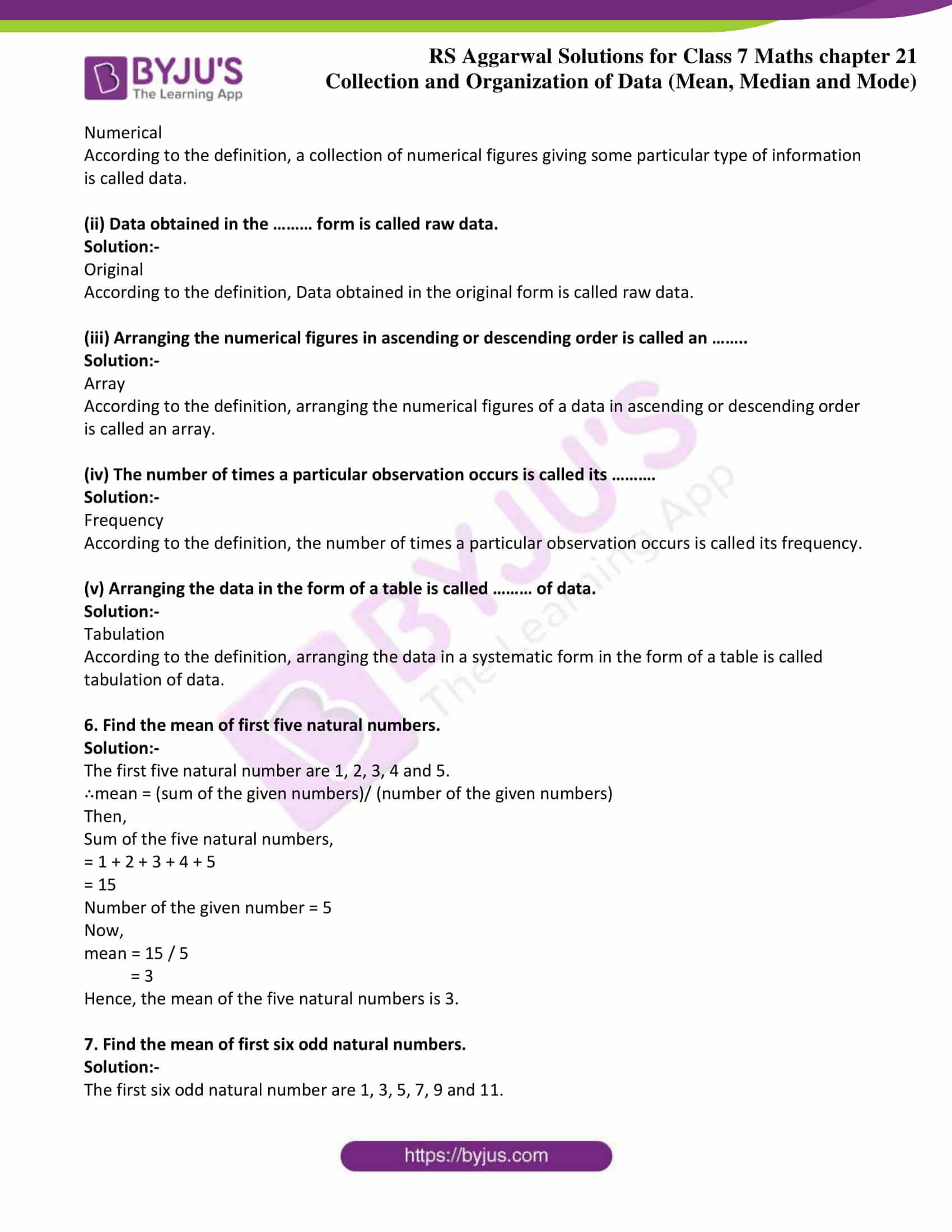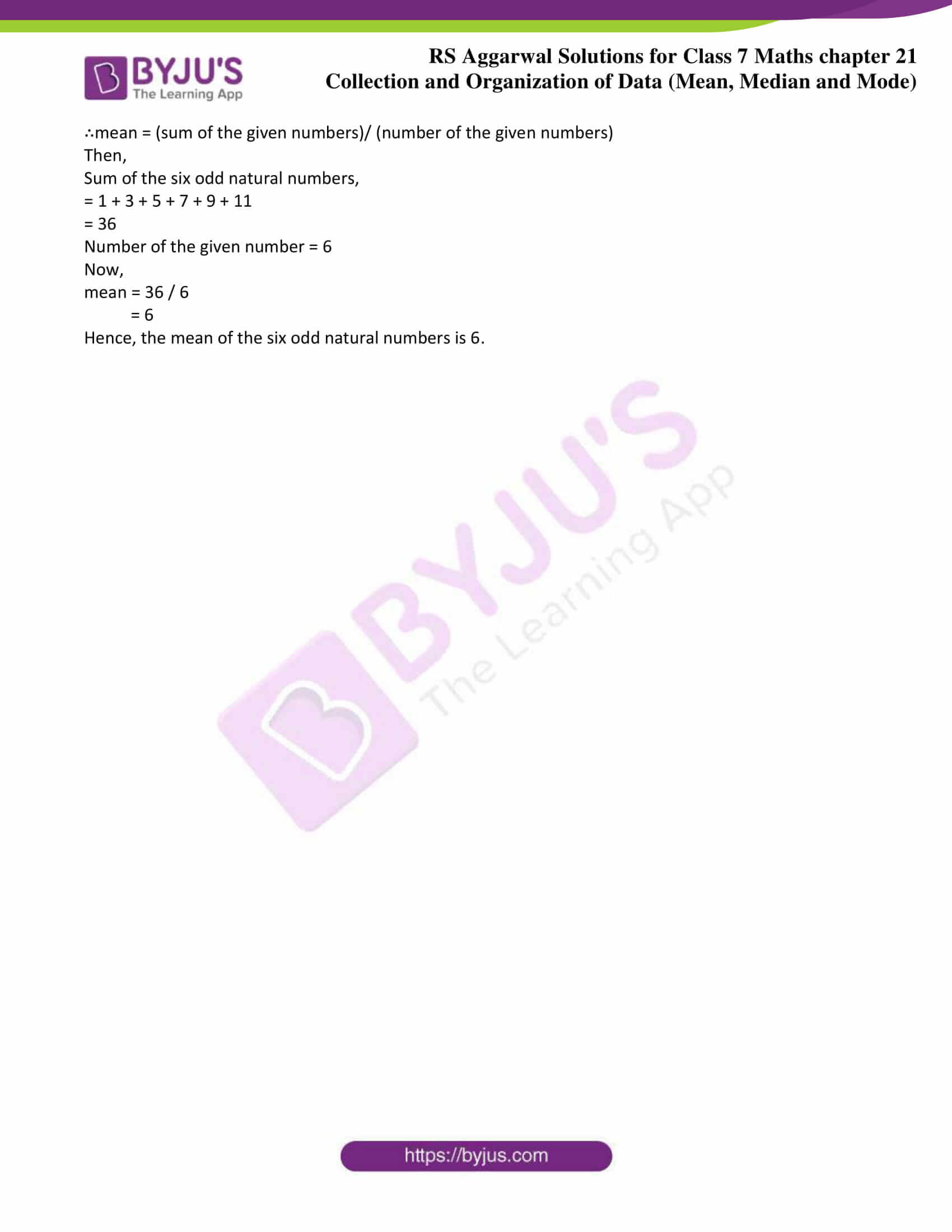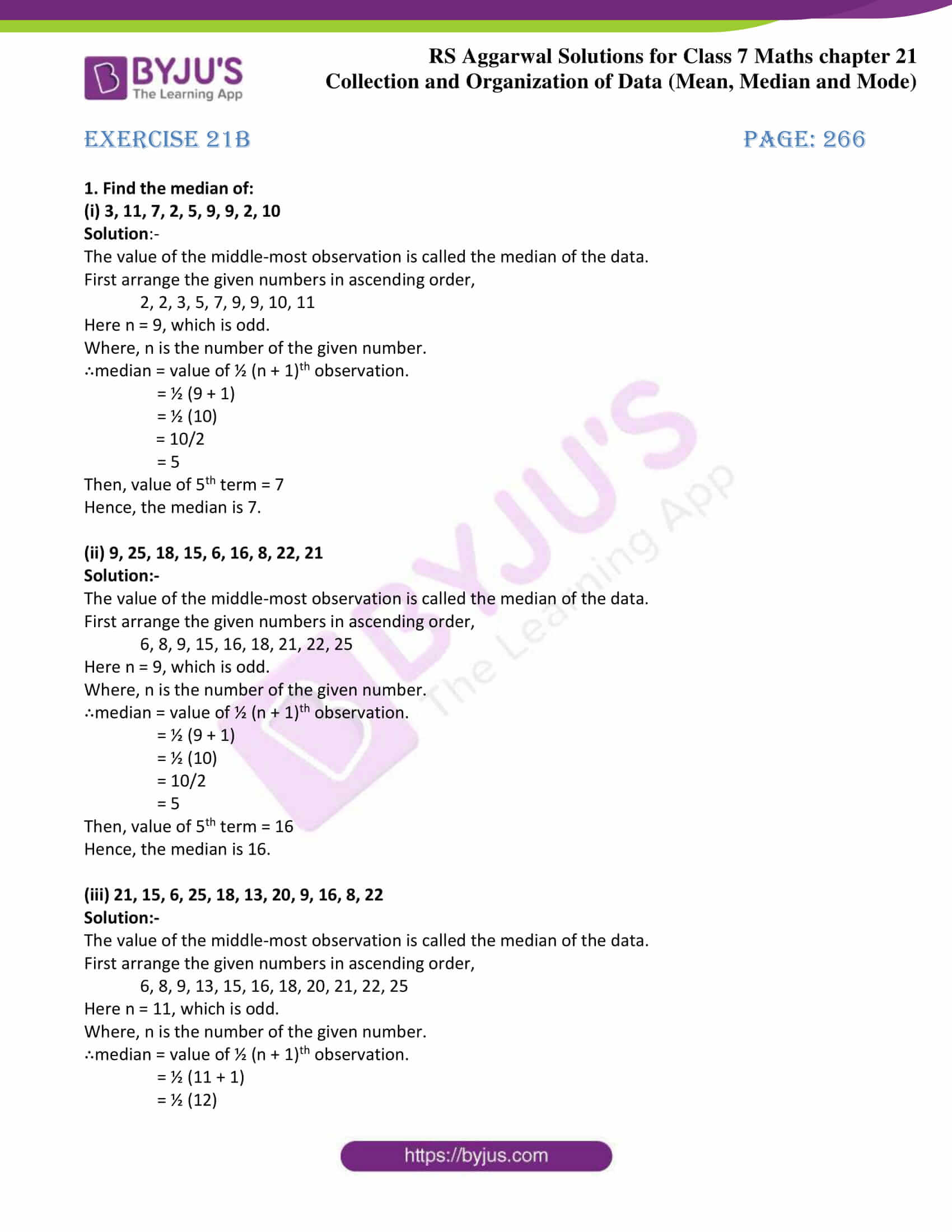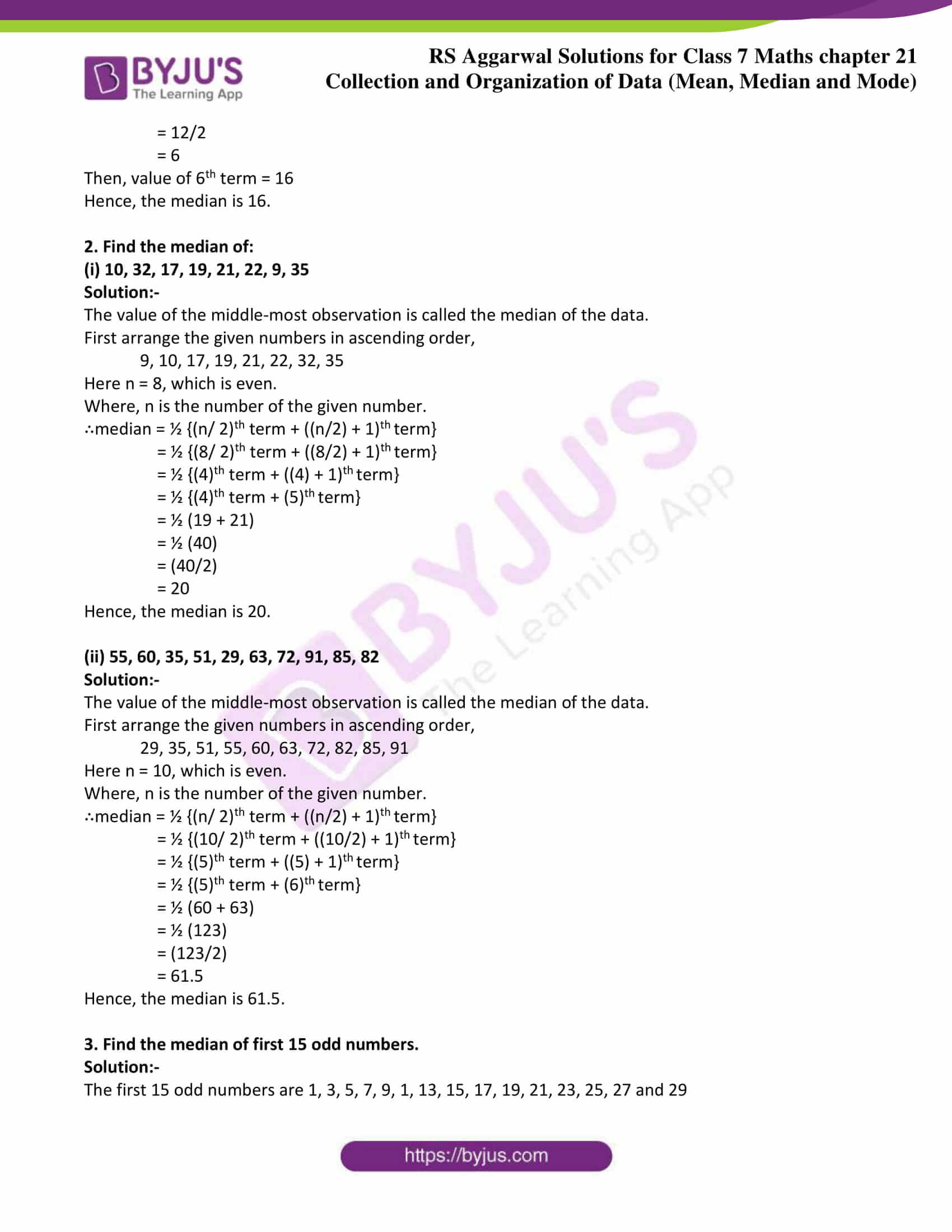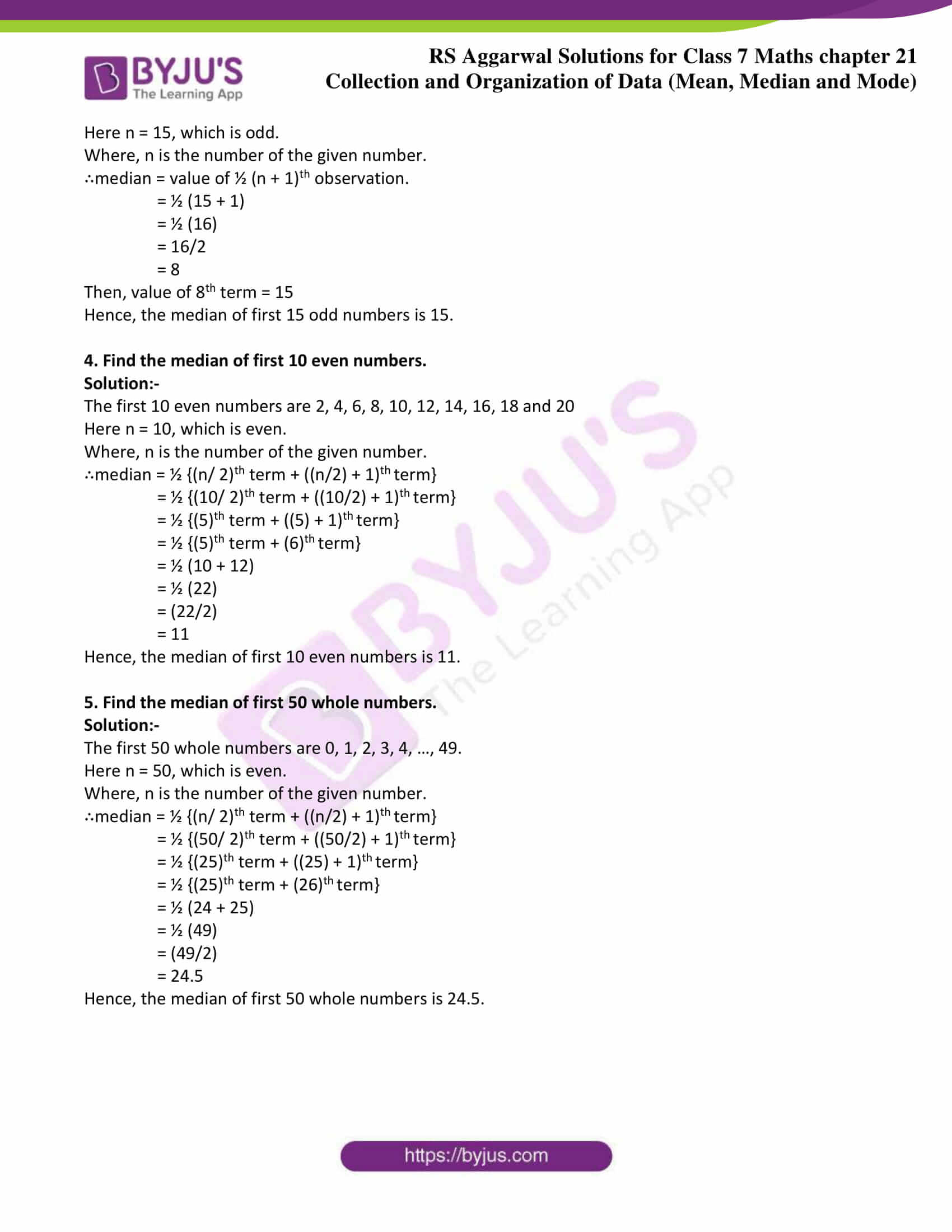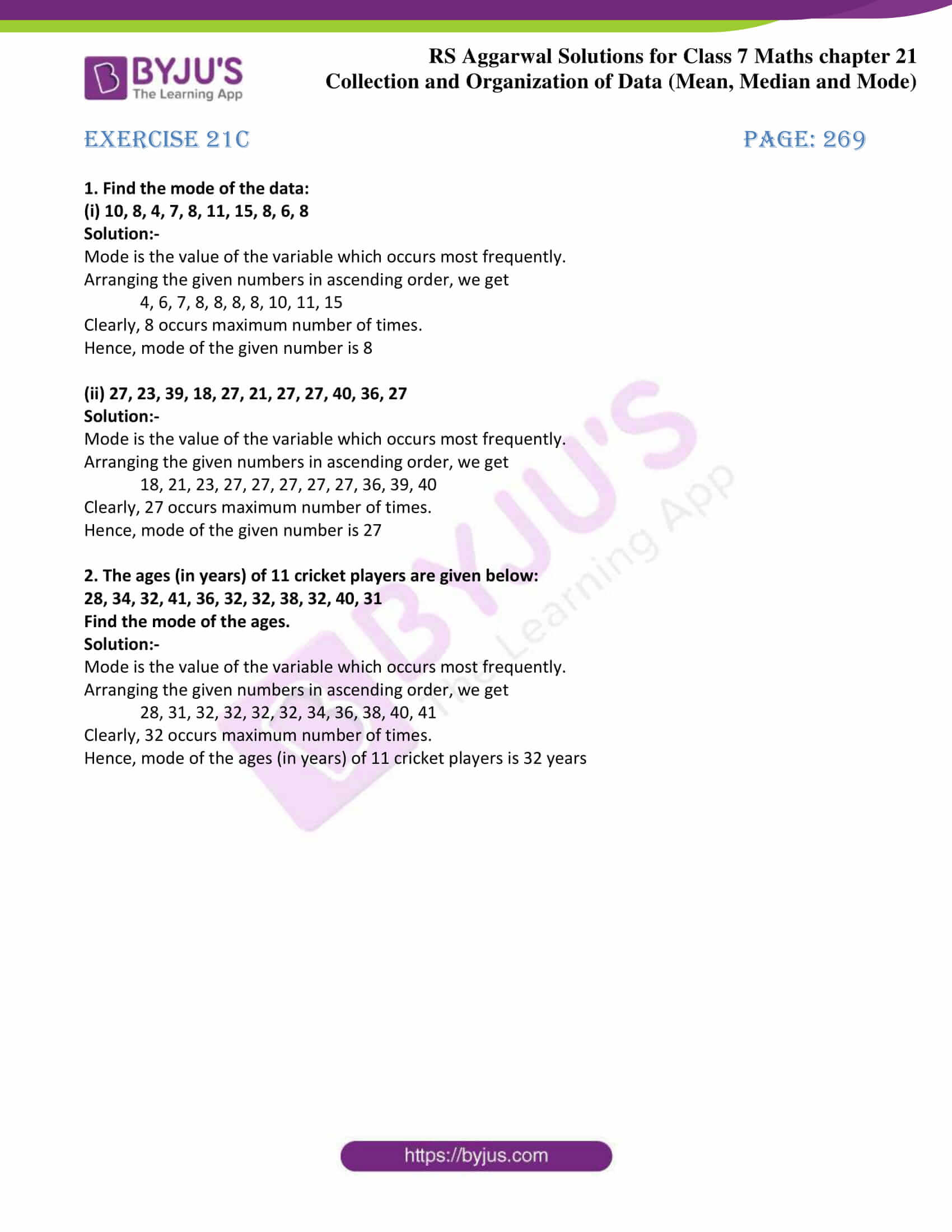Exercise 21A

Exercise 21B

Exercise 21C

## Exercise 21A Page: 263

1. Define the terms:

(i) Data

Definition:-

A collection of numerical figures giving some particular type of information is called data.

(ii) Raw data

Definition:-

Data obtained in the original form is called raw data.

(iii) Array

Definition:-

Arranging the numerical figures of a data in ascending or descending order is called an array.

(iv) Tabulation of data

Definition:-

Arranging the data in a systematic form in the form of a table is called tabulation of data.

(v) Observation

Definition:-

Each numerical figure in a data is called an observation.

(vi) Frequency of an observation

Definition:-

The number of times a particular observation occurs is called its frequency.

(vii) Statistics

Definition:-

It is the subject that deals with the collection, presentation, analysis and interpretation of numerical data.

2. A die was thrown 20 times and the following outcomes were recorded:

5, 2, 1, 3, 4, 4, 5, 6, 2, 2, 4, 5, 5, 6, 2, 2, 4, 5, 5, 1.

Arrange the above data in ascending order and prepare the frequency table.

Solution:-

Arranging the data in ascending order, we get

1, 1, 2, 2, 2, 2, 2, 3, 4, 4, 4, 4, 5, 5, 5, 5, 5, 5, 6, 6.

Now, we may prepare the frequency table of the given data, as shown below.

 Outcomes of Die Number of Outcomes (Frequency) 1 2 2 5 3 1 4 4 5 6 6 2

3. The daily wages (in ₹) 0f 15 workers in a factory are given below:

400, 360, 300, 300, 260, 360, 360, 400, 300, 260, 360, 360, 400, 300, 360

Arrange the above data in ascending order and prepare the frequency table.

Solution:-

Arranging the data in ascending order, we get

260, 260, 300, 300, 300, 300, 360, 360, 360, 360, 360 360, 400, 400, 400.

Now, we may prepare the frequency table of the given data, as shown below.

 Daily wages in ₹ Frequency 260 2 300 4 360 6 400 3

4. Write the data given below in ascending order and prepare the frequency table.

7, 8, 7, 10, 6, 8, 9, 7, 10, 5, 7, 6, 8, 5, 6, 7, 8, 9, 7, 6, 7, 8

Solution:-

Arranging the data in ascending order, we get

5, 5, 6, 6, 6, 6, 7, 7, 7, 7, 7, 7, 7, 8, 8, 8, 8, 8, 9, 9, 10, 10

 Observation Frequency 5 2 6 4 7 7 8 5 9 2 10 2

5. Fill in the blanks:

(i) Data means information in the form of …… figures.

Solution:-

Numerical

According to the definition, a collection of numerical figures giving some particular type of information is called data.

(ii) Data obtained in the ……… form is called raw data.

Solution:-

Original

According to the definition, Data obtained in the original form is called raw data.

(iii) Arranging the numerical figures in ascending or descending order is called an ……..

Solution:-

Array

According to the definition, arranging the numerical figures of a data in ascending or descending order is called an array.

(iv) The number of times a particular observation occurs is called its ……….

Solution:-

Frequency

According to the definition, the number of times a particular observation occurs is called its frequency.

(v) Arranging the data in the form of a table is called ……… of data.

Solution:-

Tabulation

According to the definition, arranging the data in a systematic form in the form of a table is called tabulation of data.

6. Find the mean of first five natural numbers.

Solution:-

The first five natural number are 1, 2, 3, 4 and 5.

∴mean = (sum of the given numbers)/ (number of the given numbers)

Then,

Sum of the five natural numbers,

= 1 + 2 + 3 + 4 + 5

= 15

Number of the given number = 5

Now,

mean = 15 / 5

= 3

Hence, the mean of the five natural numbers is 3.

7. Find the mean of first six odd natural numbers.

Solution:-

The first six odd natural number are 1, 3, 5, 7, 9 and 11.

∴mean = (sum of the given numbers)/ (number of the given numbers)

Then,

Sum of the six odd natural numbers,

= 1 + 3 + 5 + 7 + 9 + 11

= 36

Number of the given number = 6

Now,

mean = 36 / 6

= 6

Hence, the mean of the six odd natural numbers is 6.

## Exercise 21B Page: 266

1. Find the median of:

(i) 3, 11, 7, 2, 5, 9, 9, 2, 10

Solution:-

The value of the middle-most observation is called the median of the data.

First arrange the given numbers in ascending order,

2, 2, 3, 5, 7, 9, 9, 10, 11

Here n = 9, which is odd.

Where, n is the number of the given number.

∴median = value of ½ (n + 1)th observation.

= ½ (9 + 1)

= ½ (10)

= 10/2

= 5

Then, value of 5th term = 7

Hence, the median is 7.

(ii) 9, 25, 18, 15, 6, 16, 8, 22, 21

Solution:-

The value of the middle-most observation is called the median of the data.

First arrange the given numbers in ascending order,

6, 8, 9, 15, 16, 18, 21, 22, 25

Here n = 9, which is odd.

Where, n is the number of the given number.

∴median = value of ½ (n + 1)th observation.

= ½ (9 + 1)

= ½ (10)

= 10/2

= 5

Then, value of 5th term = 16

Hence, the median is 16.

(iii) 21, 15, 6, 25, 18, 13, 20, 9, 16, 8, 22

Solution:-

The value of the middle-most observation is called the median of the data.

First arrange the given numbers in ascending order,

6, 8, 9, 13, 15, 16, 18, 20, 21, 22, 25

Here n = 11, which is odd.

Where, n is the number of the given number.

∴median = value of ½ (n + 1)th observation.

= ½ (11 + 1)

= ½ (12)

= 12/2

= 6

Then, value of 6th term = 16

Hence, the median is 16.

2. Find the median of:

(i) 10, 32, 17, 19, 21, 22, 9, 35

Solution:-

The value of the middle-most observation is called the median of the data.

First arrange the given numbers in ascending order,

9, 10, 17, 19, 21, 22, 32, 35

Here n = 8, which is even.

Where, n is the number of the given number.

∴median = ½ {(n/ 2)th term + ((n/2) + 1)th term}

= ½ {(8/ 2)th term + ((8/2) + 1)th term}

= ½ {(4)th term + ((4) + 1)th term}

= ½ {(4)th term + (5)th term}

= ½ (19 + 21)

= ½ (40)

= (40/2)

= 20

Hence, the median is 20.

(ii) 55, 60, 35, 51, 29, 63, 72, 91, 85, 82

Solution:-

The value of the middle-most observation is called the median of the data.

First arrange the given numbers in ascending order,

29, 35, 51, 55, 60, 63, 72, 82, 85, 91

Here n = 10, which is even.

Where, n is the number of the given number.

∴median = ½ {(n/ 2)th term + ((n/2) + 1)th term}

= ½ {(10/ 2)th term + ((10/2) + 1)th term}

= ½ {(5)th term + ((5) + 1)th term}

= ½ {(5)th term + (6)th term}

= ½ (60 + 63)

= ½ (123)

= (123/2)

= 61.5

Hence, the median is 61.5.

3. Find the median of first 15 odd numbers.

Solution:-

The first 15 odd numbers are 1, 3, 5, 7, 9, 1, 13, 15, 17, 19, 21, 23, 25, 27 and 29

Here n = 15, which is odd.

Where, n is the number of the given number.

∴median = value of ½ (n + 1)th observation.

= ½ (15 + 1)

= ½ (16)

= 16/2

= 8

Then, value of 8th term = 15

Hence, the median of first 15 odd numbers is 15.

4. Find the median of first 10 even numbers.

Solution:-

The first 10 even numbers are 2, 4, 6, 8, 10, 12, 14, 16, 18 and 20

Here n = 10, which is even.

Where, n is the number of the given number.

∴median = ½ {(n/ 2)th term + ((n/2) + 1)th term}

= ½ {(10/ 2)th term + ((10/2) + 1)th term}

= ½ {(5)th term + ((5) + 1)th term}

= ½ {(5)th term + (6)th term}

= ½ (10 + 12)

= ½ (22)

= (22/2)

= 11

Hence, the median of first 10 even numbers is 11.

5. Find the median of first 50 whole numbers.

Solution:-

The first 50 whole numbers are 0, 1, 2, 3, 4, …, 49.

Here n = 50, which is even.

Where, n is the number of the given number.

∴median = ½ {(n/ 2)th term + ((n/2) + 1)th term}

= ½ {(50/ 2)th term + ((50/2) + 1)th term}

= ½ {(25)th term + ((25) + 1)th term}

= ½ {(25)th term + (26)th term}

= ½ (24 + 25)

= ½ (49)

= (49/2)

= 24.5

Hence, the median of first 50 whole numbers is 24.5.

## Exercise 21c Page: 269

1. Find the mode of the data:

(i) 10, 8, 4, 7, 8, 11, 15, 8, 6, 8

Solution:-

Mode is the value of the variable which occurs most frequently.

Arranging the given numbers in ascending order, we get

4, 6, 7, 8, 8, 8, 8, 10, 11, 15

Clearly, 8 occurs maximum number of times.

Hence, mode of the given number is 8

(ii) 27, 23, 39, 18, 27, 21, 27, 27, 40, 36, 27

Solution:-

Mode is the value of the variable which occurs most frequently.

Arranging the given numbers in ascending order, we get

18, 21, 23, 27, 27, 27, 27, 27, 36, 39, 40

Clearly, 27 occurs maximum number of times.

Hence, mode of the given number is 27

2. The ages (in years) of 11 cricket players are given below:

28, 34, 32, 41, 36, 32, 32, 38, 32, 40, 31

Find the mode of the ages.

Solution:-

Mode is the value of the variable which occurs most frequently.

Arranging the given numbers in ascending order, we get

28, 31, 32, 32, 32, 32, 34, 36, 38, 40, 41

Clearly, 32 occurs maximum number of times.

Hence, mode of the ages (in years) of 11 cricket players is 32 years

## RS Aggarwal Solutions for Class 7 Maths Chapter 21 – Collection and Organisation of Data (Mean, Median and Mode)

Chapter 21 – Collection and Organisation of Data (Mean, Median and Mode) contains 3 exercises and the RS Aggarwal Solutions available on this page provide solutions to the questions present in the exercises. Now, let us have a look at some of the concepts discussed in this chapter.

• Some Definitions
• Statistics
• Mean of Ungrouped Data
• Mean of Tabulated Data
• Median of Ungrouped Data
• Median of Discrete Series
• Mode of Ungrouped Data

### Chapter Brief of RS Aggarwal Solutions for Class 7 Maths Chapter 21 – Collection and Organisation of Data (Mean, Median and Mode)

RS Aggarwal Solutions for Class 7 Maths Chapter 21 – Collection and Organisation of Data (Mean, Median and Mode) is a topic in Arithmetic which is a branch of Mathematics. In this chapter, students are going to learn about mean, median and mode. These are all the concepts to measure the central tendency of the given data. This data may be related to the profits of a company during the last few years, the monthly wages earned by workers in a factory, the marks obtained by the students of a class in a particular examination etc.